# Chapter 3 Coordinate Geometry RS Aggarwal Solution for Class 9th Maths

0
1138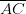Introduction toEuclid’s Geometry

# Question 1:

Exercise 3A

A theorem is a statement that requires a proof. Whereas, a basic fact which is taken for granted, without proof, is called an axiom.

Example of Theorem: Pythagoras Theorem

Example of axiom: A unique line can be drawn through any two points.

# Question 2:

1. Line segment: The straight path between two points is called a line segment.
2. Ray: A line segment when extended indefinitely in one direction is called a ray.
3. Intersecting Lines: Two lines meeting at a common point are called intersecting lines, i.e., they have a common point.
4. Parallel Lines: Two lines in a plane are said to be parallel, if they have no common point, i.e., they do not meet at all.
5. Half-line: A ray without its initial point is called a half-line.
6. Concurrent lines: Three or more lines are said to be concurrent, if they intersect at the same point.
7. Collinear points: Three or more than three points are said to be collinear, if they lie on the same line.
8. Plane: A plane is a surface such that every point of the line joining any two points on it, lies on it.

# Question 3:

1. Six points: A,B,C,D,E,F
2. Five line segments: , , , ,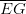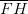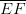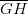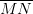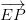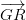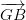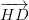1. Four rays: , , ,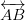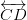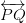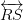1. Four lines: , , ,
2. Four collinear points: M,E,G,B

# Question 4: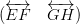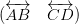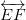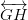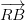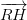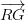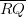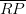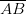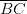1. and their corresponding point of intersection is R. and their corresponding point of intersection is P.
2. , , and their point of intersection is R.
3. Three rays are: , ,
4. Two line segments are: ,

# Question 5:

1. An infinite number of lines can be drawn to pass through a given point.
2. One and only one line can pass through two given points.
3. Two given lines can at the most intersect at one and only one point.
4. , ,

1. False
2. False
3. False
4. True
5. False
6. True
7. True
8. True
9. True
10. False
11. False
12. True